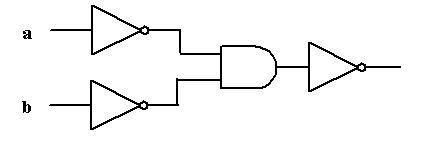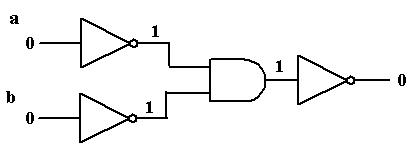Lecture Circuits 3
Analyzing a Circuit

Look at the following circuit.  What does it do?Let's build a logic table and see!  We want to see what the output is for all combinations of input values:
 a      b output 0        0 ?? 0        1 ?? 1        0 ?? 1        1 ??

First, let's see what we get if both a=0 and b=0, by tracing what happens on the circuit diagram:So we have, so far:
 a      b output 0        0 0 0        1 ?? 1        0 ?? 1        1 ??

By repeating the above for (1)  a=0 and b=1 (2) a=1 and b=0  (3) a=1 and b=1,  our completed table becomes:

 a      b output 0        0 0        1 1        0 1        1

Does this logic table look like that of a gate we already know?  Click here for the exciting answer!

It's the logic table for the OR gate!  This proves that (theoretically) we really don't need OR;  we can build it out of AND and NOT!

 Anything any computer can do anywhere, anytime, can be done with 0's, 1's,  AND gates and NOT gates Remarkable!